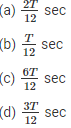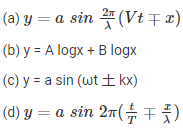# Oscillations and Waves MCQ for NEET

NEET  Physics is the scoring paper in the medical entrance examination. Here, you will discover the NEET Physics MCQ Questions for all Concepts as per the latest syllabus. Practice more on a regular basis with these NEET Physics objective questions on air pollution and improve your subject knowledge & problem-solving skills along with time management. NEET Physics Oscillations and Waves Multiple Choice Questions make you feel confident in answering the question in the exam & increases your scores to high.

## MCQ on Oscillations and Waves

1. Which of the following graph depicts the variation in the potential energy for a particle executing SHM at the origin x = 0?2. Which of the following graph represents the variation in the potential energy as a function of time t and displacement x if the displacement of the particle is x = A cos⍵t(a)I,IV
(b) II, III
(c) I,III
(d) II,IV

3. What is the time taken by a particle executing SHM with a time period T sec from a positive extreme position to half of the amplitude?Answer: (a) $$\frac{2 T}{12} \mathrm{sec}$$

4. On which of the following does the energy of a simple harmonic motion depend
(a) ⍵
(b) $$\frac{1}{\omega^{2}}$$
(c) $$\frac{1}{a^{2}}$$
(d) a2

5. Calculate the amplitude for a SHM using the equation x = 3sin2pt + 4cos3pt
(a) 3
(b) 5
(c) 4
(d) 7

6. What is the constant of propotionality of an oscillator if the damping force is directly propotional to the velocity
(a) kg.s-1
(b) kg.m.s-1
(c) kg.s
(d) kg.m.s-2

7. Choose the equation that cannot represent a plane progressive waveAnswer: (b) y = A logx + B logx

8. What is the phase difference between the prongs of the tuning fork
(a) 5π
(b) π
(c) 2π
(d) 3π

9. What is the change in the phase if a wave is reflected from a denser medium
(a) 3π
(b) 0
(c) π
(d) 2π

10. The observer hears a sound from the source which is moving away from the observer who is stationary. Determine the frequency of the sound
(a) It is halved
(b) It remains the same
(c) It reaches infinity
(d) It gets doubled

11. The length of the pendulum which is displaying SHM is increased by 21%. Calculate the percentage increase in the periodic time of the pendulum.
(a) 21%
(b) 10%
(c) 11%
(d) 42%